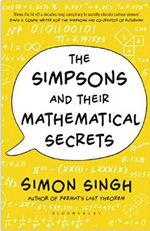Plus Advent Calendar Door #9: Mathematical Simpsons

Plus Advent Calendar Door #9: Mathematical SimpsonsIf you've got maths friends then you might have noticed that a large subset of them are also Simpsons fans. And there's a good reason for that: as Simon Singh's book The Simpsons and their mathematical secrets explains, a large number of Simpsons writers are mathematicians. The book explores the maths that has been hidden (and not so hidden) throughout the series and will make a great present for maths/Simpsons nerds.